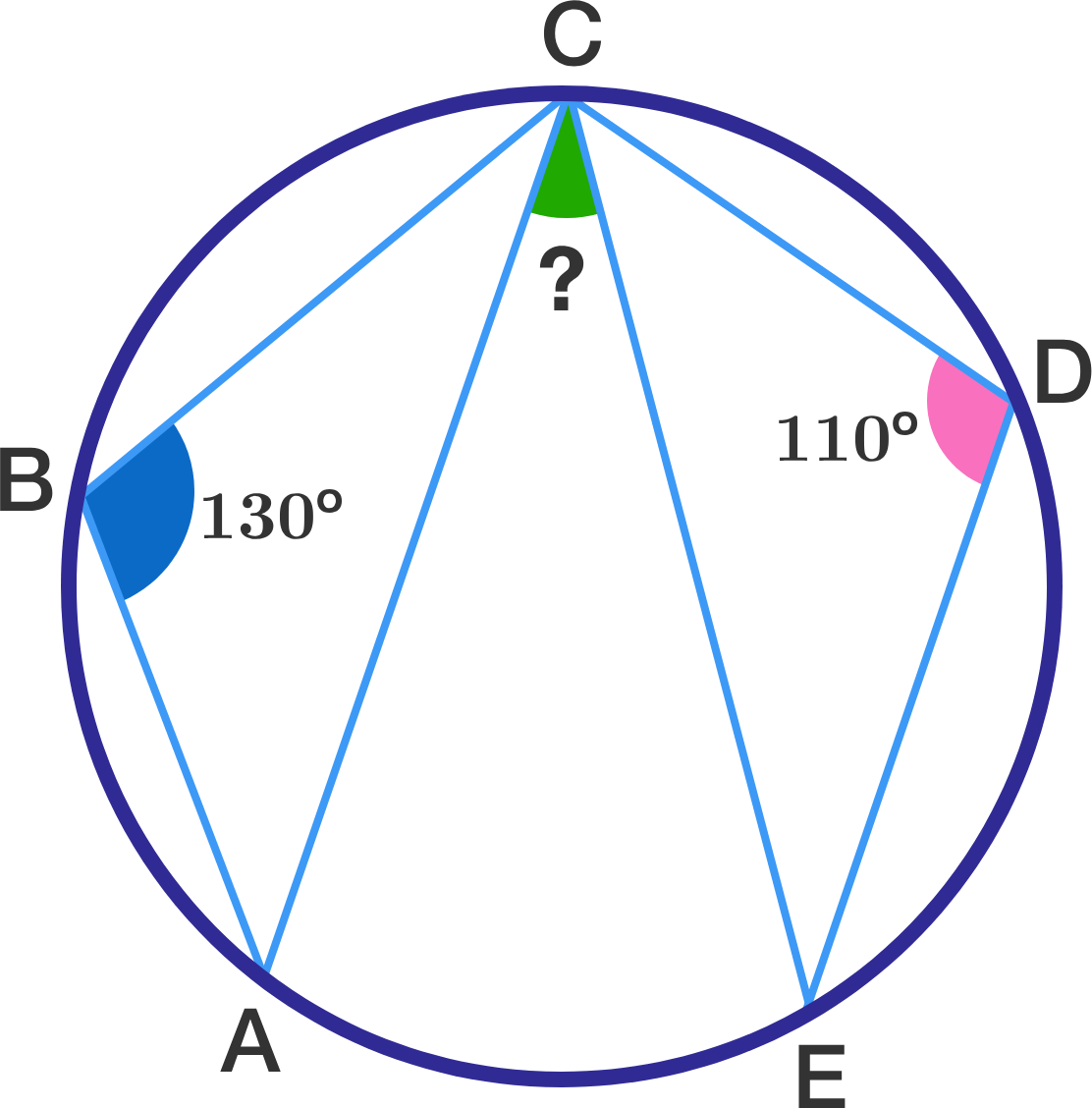KVPY 2016 SA Question 6

Geometry Level 1The points $A$, $B$, $C$, $D$, and $E$ are marked on the circumference of a circle in order such that $\angle ABC = 130^\circ$ and $\angle CDE = 110^\circ$.

Find the measure of the green angle, $\angle ACE$, in degrees.

Note: The diagram is not necessarily up to scale.

×